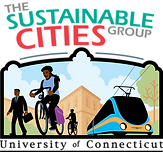###### due Wed March 13##### Question 1

###### ​##### Question 2
###### ​

Sketch the network described by the accompanying link table.

Find by observation the 'minimum tree' from node 1 and 2, respectively.

Make a Tree Table for the 'minimum tree' for node 1.

##### Question 3
###### ​

Based on the tree table given, sketch the minimum tree for zone 2.

The peak hour interchange volumes between Zone 2 and the other zones in the study are given below.  Use a Free-flow/All-or-Nothing procedure to allocate the traffic to the links in the network.

##### Question 4
###### (Ex. 4 pg. 129, Papacostas and Prevedouros)

Given s = 0.30 / (60 - u), derive the relationships u-k, u-q, and q-k.

Estimate the maximum theoretical flow of the roadway.

Note: s - spacing in miles, u - speed in miles per hour (mph)

.

##### Question 5
###### (Ex. 8 pg. 129, Papacostas and Prevedouros)

A study of traffic using a bridge showed that the speed-concentration relationship is

u = 17.2 ln (228/k)

Find the a) free-flow speed (uf), b) the theoretical maximum flow on the bridge (qmax), c) the speed (um) and concentration (km) that correspond to the maximum flow, and d) the jam concentration (kj).

Note: k - concentration in veh/mi, u - speed in miles per hour (mph)

.

##### Question 6
###### ​

Six vehicles run time trials on a 1 mile track.

The speed for the six vehicles are 10, 20, 15, 16, 25 and 22 mph, respectively.

1. Calculate TMS and SMS based on these speeds.

2. Which is the correct measure of mean speed in this situation?  TMS or SMS.

Verify that your answer to 2 above is correct by determining the mean speed from first principles.  In other words, calculate the mean speed by the total travel distance and travel time for the six vehicles combined.